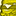# QlikView App Dev

Discussion Board for collaboration related to QlikView App Development.

Announcements
QlikWorld 2023, a live, in-person thrill ride. Save \$300 before February 6: REGISTER NOW!
cancel
Showing results for
Did you mean:Contributor II

## Create QlikSense Table Showing counts for NULL, Total Rows, % NULL

Hello,

I have the data in following table that am trying to replicate in Qlik Sense the calculations I perform in Excel to count the total number of rows of data for each data item as well as the total number of NULL values in each column.

In excel for each column I would use the following calculations:

 Count NULL = COUNTBLANK(Column_X) Count NOT NULL = COUNTA(Column_X) Total Count = (Count NULL) + (Count NOT NULL) % NULL = Count NULL/TotalCount

I have attached a file Qlik_NULL_Count_Test_Data.csv  with sample data that shows the values I am wishing to calculate and display.

How would I do this in Qlik Sense?

Many thanks

1 Solution

Accepted SolutionsContributor III

Hi Matthew,

There is a concept called, "Cross table", you can use here.

NullTest:

CrossTable(Fields, Data)

ID,

[Start Date],

[End Date],

[Number of Cycles],

Protocol,

[Drug Name],

Dose,

Route,

Frequency,

[Frequency Unit],

Day,

[Cycle Number],

[Cycle Start Date],

[Cycle End Date]

FROM [lib://DEMO/Qlik_NULL_Count_Test_Data.csv]

(txt, codepage is 28591, embedded labels, delimiter is ',', msq);

Once reload Done. Take Table Chart use following dimension & expression

Dimension:

Parameter => Fields

Measure:

Total Null =>  Sum(If(Data='',1,0))

Total Not Null => Sum(If(Data='',0,1))

Total =>  Count(Data)

OR

Sum(If(Data='',1,0))  Sum(If(Data='',0,1))

% OF Null => Num(Sum(If(Data='',1,0))/Count(Data),'#,#0.0%')    <<== Final Result

Data Validation also done . please find screenshot for reference.Note: In CSV file, which you have provided, For Some Column Count Not Null is not correct, For an example : For Not Null Count for Drug Name, End Date, Route, Day, instead of COUNTA(), Count () function has been used, I think. Other wise, everything validated.

I hope, it will work for you, If it works, mark as correct answer, so that others can also direct reach to answer.

2 RepliesContributor III

Hi Matthew,

There is a concept called, "Cross table", you can use here.

NullTest:

CrossTable(Fields, Data)

ID,

[Start Date],

[End Date],

[Number of Cycles],

Protocol,

[Drug Name],

Dose,

Route,

Frequency,

[Frequency Unit],

Day,

[Cycle Number],

[Cycle Start Date],

[Cycle End Date]

FROM [lib://DEMO/Qlik_NULL_Count_Test_Data.csv]

(txt, codepage is 28591, embedded labels, delimiter is ',', msq);

Once reload Done. Take Table Chart use following dimension & expression

Dimension:

Parameter => Fields

Measure:

Total Null =>  Sum(If(Data='',1,0))

Total Not Null => Sum(If(Data='',0,1))

Total =>  Count(Data)

OR

Sum(If(Data='',1,0))  Sum(If(Data='',0,1))

% OF Null => Num(Sum(If(Data='',1,0))/Count(Data),'#,#0.0%')    <<== Final Result

Data Validation also done . please find screenshot for reference.Note: In CSV file, which you have provided, For Some Column Count Not Null is not correct, For an example : For Not Null Count for Drug Name, End Date, Route, Day, instead of COUNTA(), Count () function has been used, I think. Other wise, everything validated.

I hope, it will work for you, If it works, mark as correct answer, so that others can also direct reach to answer.Contributor II
Author

Thank you Amit,

that seems to have worked. I'vemarked the answer as correct.

A further question; My load statement loads data from many unique tables holding the same data, is it possible to simply add the CrossTable and LOAD statements to each existing unique table load?

CrossTable(Fields, Data)